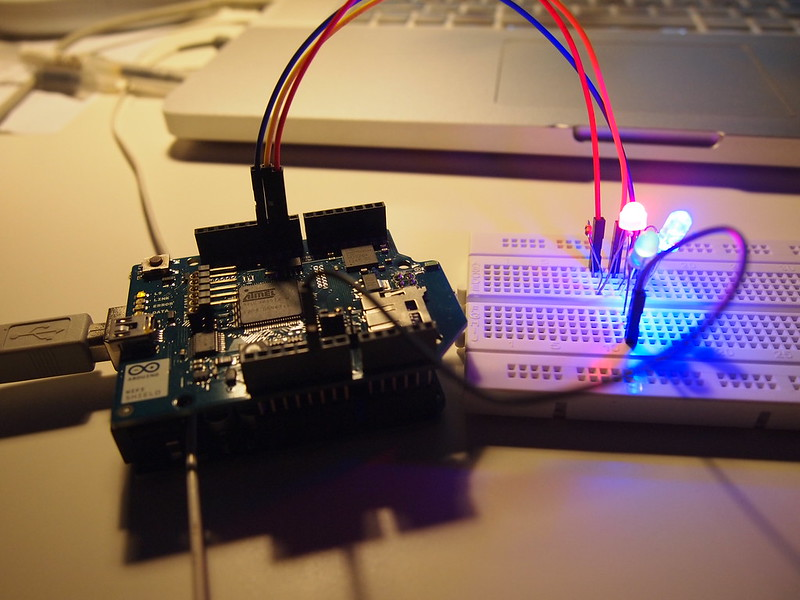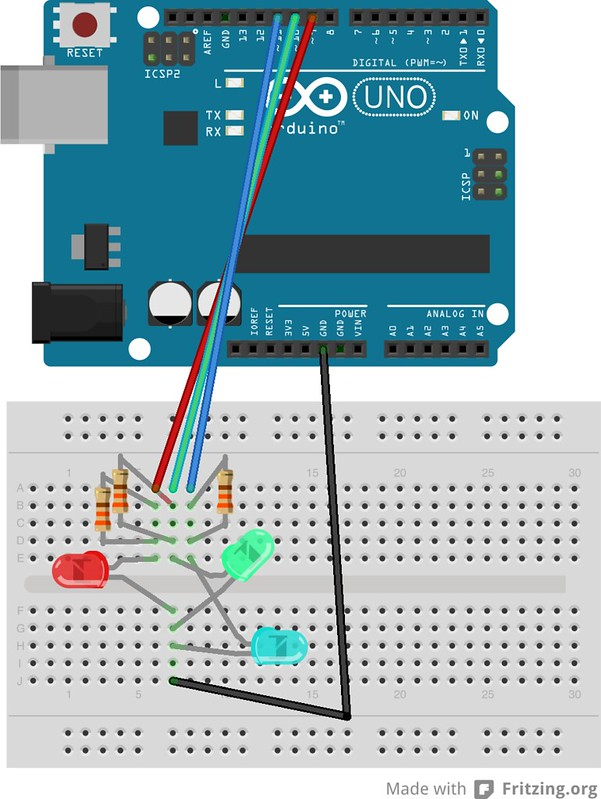# Simple Colour-changing Ambient Lamp (Arduino + 3 LED)In this continuation of posts on learning to do the most basic of things with the Arduino, the next experiment I made was a colour-changing lamp with 3 different LEDs. I followed this simple instructable but edited slightly. I noticed there were lots of “#define” in the instructable – it seems that #define is something from C that is for defining a constant. What is the difference between using #define and int to define which pins each LED is controlled via? Well in practice nothing much seems to be different except the conceptual difference is that #define is for defining a CONSTANT whereas technically speaking an int would be initialising a VARIABLE that can be changed later on in the program, although in this case we don’t really intend to change the pin number. But we could, if we wanted to.

Things with # in front of them like #include and #define are preprocessed before the program compiles. Also, apparently #define does not take up any program space, whereas int is stored as variable in RAM. For more info see this wiki entry on C Preprocessor.

# Colour Changing Lamp

For this you’ll need: an arduino uno, 4 jumper wires, 3 LEDs of different colour, and 3 x 330ohm resistors

```int delayTime = 2;
int maxBrightness = 255;
int redPin = 9;
int greenPin = 10;
int bluePin = 11;
int red = 0;
int green = 170;
int blue = 170;```

// Indicates whether a color will be incrementing (1) or decrementing (0) in brightness
int incR = 1;
int incG = 1;
int incB = 0;

void transition()
{
if (red >= maxBrightness)
incR = 0;
else if (red <= 0)
incR = 1;
if (green >= maxBrightness)
incG = 0;
else if (green <= 0)
incG = 1;
if (blue >= maxBrightness)
incB = 0;
else if (blue <= 0)
incB = 1;

if (incR)
red++;
else
red–;
if(incG)
green++;
else
green–;
if(incB)
blue++;
else
blue–;
}

void setColor()
{
analogWrite(redPin, red);
analogWrite(greenPin, green);
analogWrite(bluePin, blue);
}

void setup()
{
// do nothing
}

void loop()
{
transition();
setColor();
delay(delayTime);
}# Stopping an Arduino Sketch

Now that you have this running, you might want to know how to make sketches stop. This baffled me for some time. My solution was eventually to create a blank sketch and to run it.

```void setup(){}
void loop(){}```

Posted on Categories Uncategorized